• Matlab升级以后，功能的确丰富了好多，有些函数也...下面我们说说，MATLAB初始化initializing极慢解决方法，但是可能导致Matlab启动的初始化很慢原因很多，这里我们只能对常见的集中可能的原因进行分析：一、Lice...

Matlab升级以后，功能的确丰富了好多，有些函数也更加人性话了，好多bug也没有了。但是可恼的是，这个该死的家伙却一天比一天肥，MATLAB7.6a居然达到了3.76G，启动和打开某些窗口，速度明显不行了，真是尾大不掉呀。

下面我们说说，MATLAB初始化initializing极慢解决方法，但是可能导致Matlab启动的初始化很慢原因很多，这里我们只能对常见的集中可能的原因进行分析：

问题原因：

解决方法：

方法一

windows

(1)右击【我的电脑】选择【属性】

(2)在【高级】选项卡中，点击【环境变量】

(5)重启Matlab

Unix,Linux,or Mac

Check to see if there is an environment variable set. Where this is

located depends on the shell you are using. Look in your home

folder. This file may be called: .cshrc (c-shell), .profile (bash),

or .bashrc (bash)

You can also create an environment variable for that shell session

from the command line using export or setenv (depending on your

shell), but the above file needs to be edited for a permanent

change.

For example:

复制代码

方法二

windows

(1)右击Matlab快捷方式，选择【属性】

(2)在【目标】中，键入如下内容

"$MATLAB\bin\win32\MATLAB.exe" -c " the MATLAB license file including file name>" 复制代码 其中$MATLAB是Matlab安装根目录，可以在Matlab中键入matlabroot得到，

(3)重启Matlab

Unix,Linux,or

MacUse a -c switch to point directly at the

If the MATLAB installer has created symbolic links, run

matlab -c

including file name>

复制代码

If symbolic links were not created, run from $MATLAB/bin/ ./matlab -c file including file name> 复制代码 Where$MATLAB is the root MATLAB directory and

to the MATLAB license file> is the absolute path to

your license file, likely in your $MATLAB/licenses directory. 二、preferences方面原因 问题原因： preferences首选参数设置的不得当，或者文件太大，Matlab启动的时候加载preferences设置需要较长时间 解决方法： 进行如下操作，需要确保您有查看隐藏文件的权限 windows (1)退出Matlab，一定要确保Matlab不再运行 (2)进入 c:\Documents and Settings\ username>\Application Data\MathWorks\MATLAB\ 复制代码 其实该路径可以在Matlab中键入prefdir得到 (3)将上述路径中，以Matlab版本号为文件名(比如R2008a)的文件夹，重命名为R2008a_old(其实这一步是在备份preferences文件) (4)重启Matlab，此时predir下会重新创建一个R2008a文件夹，里面包含了所有的preferences设置，只是属性值都是默认的，当然以前对Matlab的设置全部没有了 (5)如果觉得不满意，那么将新生成的R2008a删除，将R2008a_old重新改为R2008a就可以了 Linux, Unix, or Mac 1) Quit MATLAB. Ensure that MATLAB is no longer running. 2) Rename the ".matlab/" directory in the users home directory, to ".matlab_old/". 3) Restart MATLAB. 三、其他方面原因 1、Matlab7.0(R14) for windows 由于部分显卡的图形库对内存使用效率不高，导致Matlab7.0长时间多频率的调用虚拟内存，具体参见http://www.matlabsky.com/thread-831-1-1.html 2、Matlab7.4(R2007a) for windoes，由于存在Matlab自身的bug导致Matlab没法启动或者长时间处于初始化状态，参见 http://www.matlabsky.com/thread-833-1-1.html 3、有部分用户反应杀毒软件会减慢Matlab运行，此时我们将Matlab的安装路径排除在杀毒软件的主动扫描范围内 展开全文• 初始化时间的原因是软件查找授权文件时间太，也就是查找许可证文件过程太费时。 （1）首先，找到安装目录下的license文件 我是严格按照安装链接的安装步骤下载的，那么此时我的license文件位于：D:\softw. Matlab2018a安装 初始化时间过长的问题解决办法： 完成安装后，电脑上我的Matlab2018a的安装地址是：D:\software\matlab2018 初始化时间过长的原因是软件查找授权文件时间太长，也就是查找许可证文件过程太费时。 （1）首先，找到安装目录下的license文件 我是严格按照安装链接的安装步骤下载的，那么此时我的license文件位于：D:\software\matlab2018\licenses\license_standalone.lic（2）打开桌面Matlab快捷方式，右键属性，在“目标”一栏的.exe后添加 " -c D:\software\matlab2018\licenses\license_standalone.lic",此处注意空格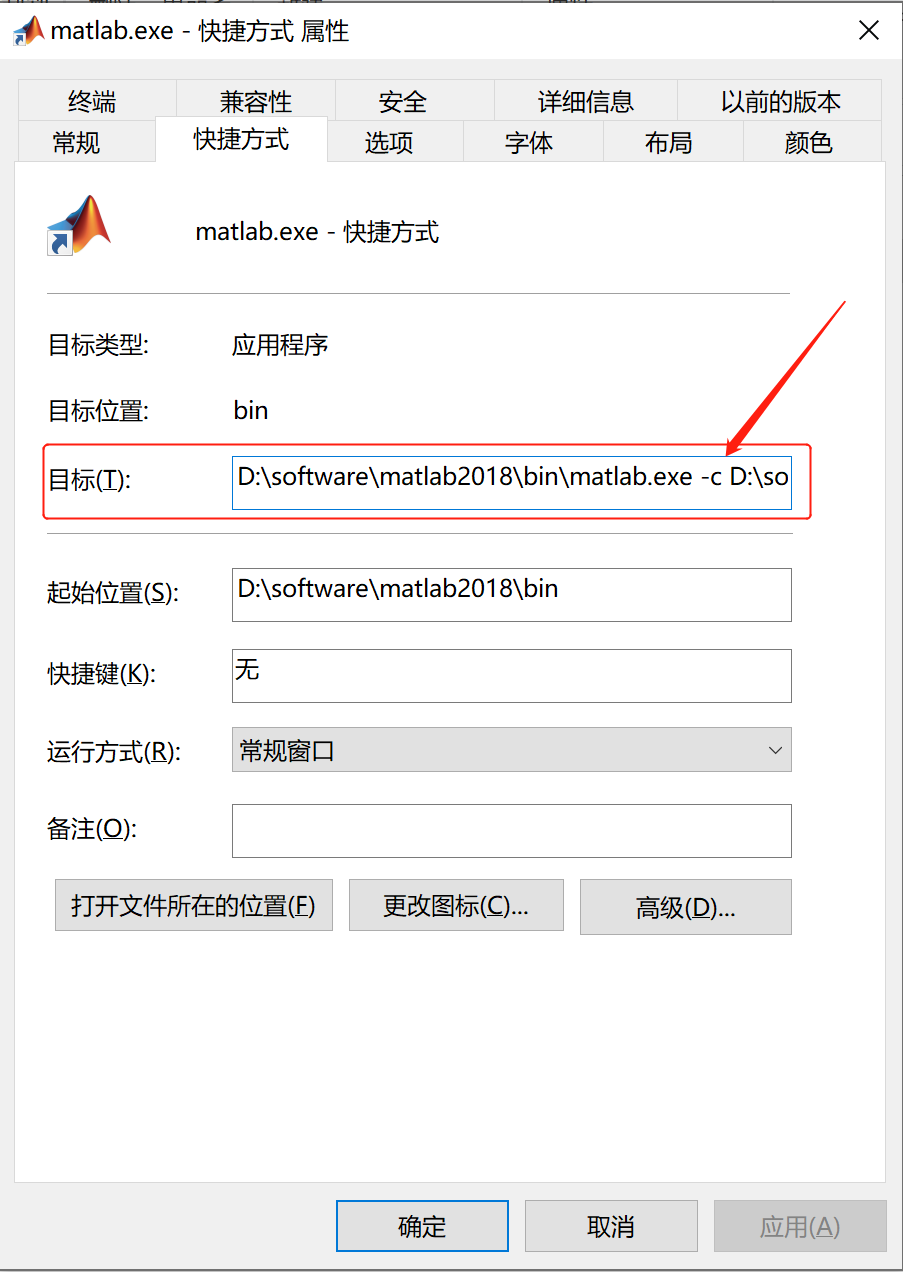（3）重启Matlab，此时打开后，初始化时间过长问题将解决。 展开全文matlab windows • 1.Matlab初始化时间 最近在另外一台电脑上重新安装了matlab，发现其初始化时间很，长达几分钟。 这里找到了两种解决办法提供参考， 共同点：找到先前matlab所使用的证书如license_server.lic和license_... 1.Matlab初始化时间过长 最近在另外一台电脑上重新安装了matlab，发现其初始化时间很长，长达几分钟。 这里找到了两种解决办法提供参考， 共同点：找到先前matlab所使用的证书如license_server.lic和license_standalone.lic所在目录 a. 加在你启动的matlab快捷方式，点击属性，找到快捷方式下的目标，添加下面的字符 -c "路径\license_server.lic" 两个证书，大部分人说加上第二个，但是在我的电脑上，加上第一个才有效。。记住一定要有冒号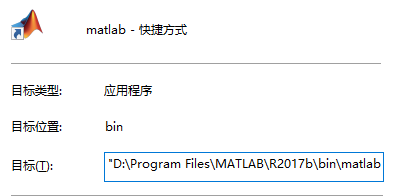b. 就是将以上我们找到的目录，在系统的环境目录中添加变量即可。 两种方法可能都有效，可能都没效果，因电脑而异 2. Office 使用（KMS工具无效情况下） 这种方法应该同样适用于之前的Office版本。 同样，之前在先前的电脑上，使用KMS工具就可以完成对Office（包括windows系统）的使用时间延长。但是最近在用另外一个电脑的时候，KMS无效。 这里提供另外一种方法，亲测有效 @echo off (cd /d "%~dp0")&&(NET FILE||(powershell start-process -FilePath '%0' -verb runas)&&(exit /B)) >NUL 2>&1 title Office 2019 Activator r/Piracy echo Converting... & mode 40,25 (if exist "%ProgramFiles%\Microsoft Office\Office16\ospp.vbs" cd /d "%ProgramFiles%\Microsoft Office\Office16")&(if exist "%ProgramFiles(x86)%\Microsoft Office\Office16\ospp.vbs" cd /d "%ProgramFiles(x86)%\Microsoft Office\Office16")&(for /f %%x in ('dir /b ..\root\Licenses16\ProPlus2019VL*.xrm-ms') do cscript ospp.vbs /inslic:"..\root\Licenses16\%%x" >nul)&(for /f %%x in ('dir /b ..\root\Licenses16\ProPlus2019VL*.xrm-ms') do cscript ospp.vbs /inslic:"..\root\Licenses16\%%x" >nul) cscript //nologo ospp.vbs /unpkey:6MWKP >nul&cscript //nologo ospp.vbs /inpkey:NMMKJ-6RK4F-KMJVX-8D9MJ-6MWKP >nul&set i=1 :server if %i%==1 set KMS_Sev=kms7.MSGuides.com if %i%==2 set KMS_Sev=kms8.MSGuides.com if %i%==3 set KMS_Sev=kms9.MSGuides.com cscript //nologo ospp.vbs /sethst:%KMS_Sev% >nul echo %KMS_Sev% & echo Activating... cscript //nologo ospp.vbs /act | find /i "successful" && (echo Complete) || (echo Trying another KMS Server & set /a i+=1 & goto server) pause >nul exit 复制上面的代码到一个txt文件，然后改后缀为bat文件，然后双击运行，出现黑框，等待黑框中显示 Complete 即可。 见下图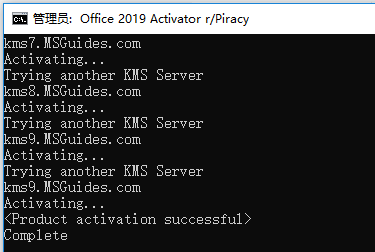win10中改后缀不是很清楚的，可以参考下面的图展开全文• 系统为Windows 10，安装完Matlab R2017a后，启动时界面左下角一直显示正在初始化：在长达十几分钟的等待以后终于初始化完成，却又出现了如下错误：... 系统为Windows 10，安装完Matlab R2017a后，启动时界面左下角一直显示正在初始化：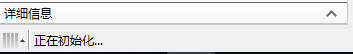在长达十几分钟的等待以后终于初始化完成，却又出现了如下错误： com.mathworks.jmi.MatlabException: Did not start the server. Desired port was: 31415. at com.mathworks.jmi.NativeMatlab.SendMatlabMessage(Native Method) at com.mathworks.jmi.NativeMatlab.sendMatlabMessage(NativeMatlab.java:259) at com.mathworks.jmi.MatlabLooper.sendMatlabMessage(MatlabLooper.java:120) at com.mathworks.jmi.Matlab.mtFeval(Matlab.java:1713) at com.mathworks.jmi.Matlab.mtEval(Matlab.java:1647) at com.mathworks.jmi.Matlab.mtEval(Matlab.java:1628) at com.mathworks.matlabserver.connector.impl.AutoStartImpl$2.run(AutoStartImpl.java:63)
at com.mathworks.matlabserver.connector.impl.AutoStartImpl$TaskListener.mvmChanged(AutoStartImpl.java:200) at com.mathworks.matlabserver.connector.impl.AutoStartImpl$TaskListener.mvmChanged(AutoStartImpl.java:182)
>>

通过网络查找解决办法以后基本锁定问题出现原因为防火墙，所以只要在防火墙设置中添加Matlab为允许通过应用就好了！具体操作如下:

1、打开Winsows设置界面，找到更新和安全。2、找到Windows安全，再找到防火墙。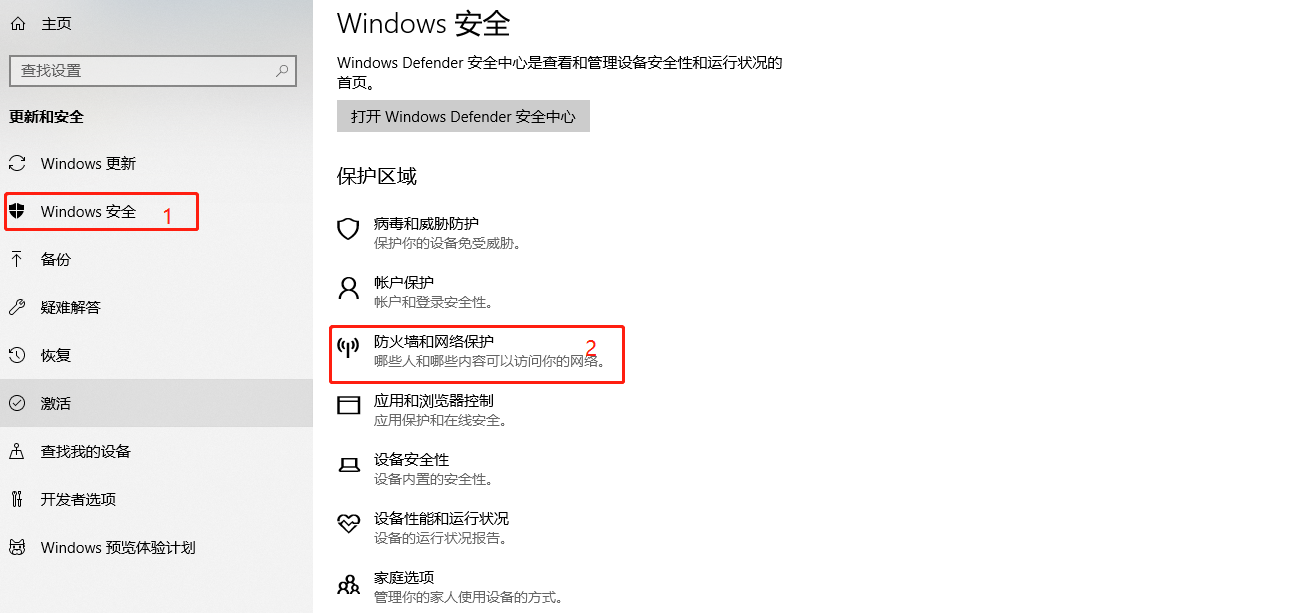3、在防火墙和网络保护中点击允许应用通过防火墙。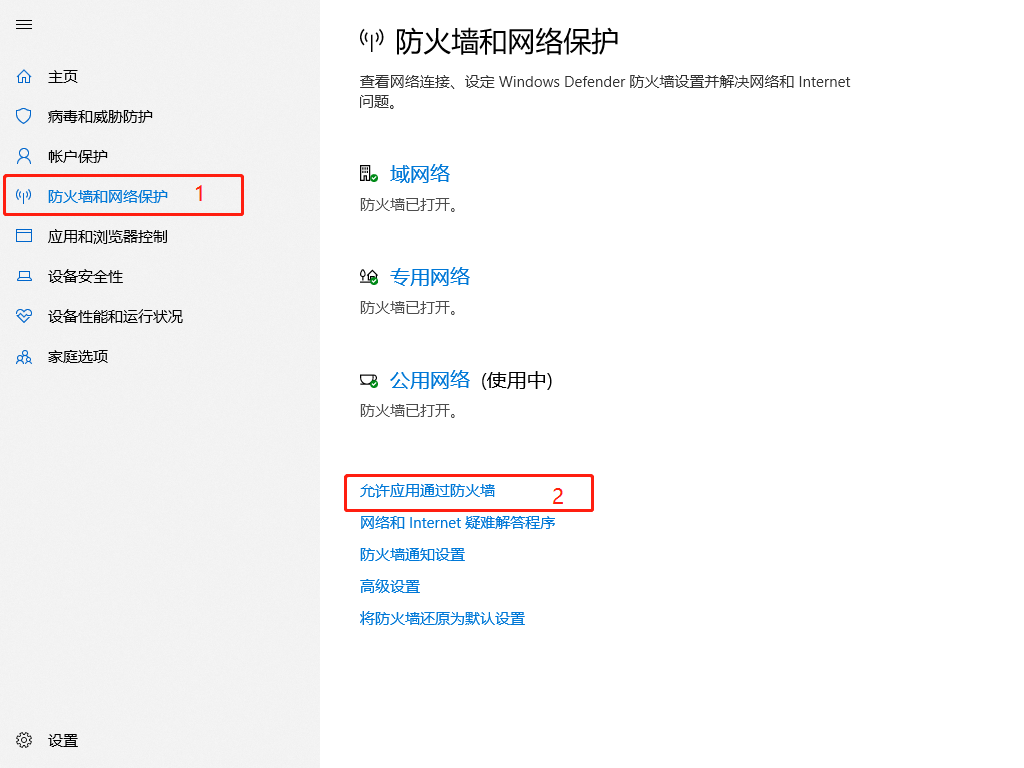4、在弹出的界面中添加Matlab应用就行了。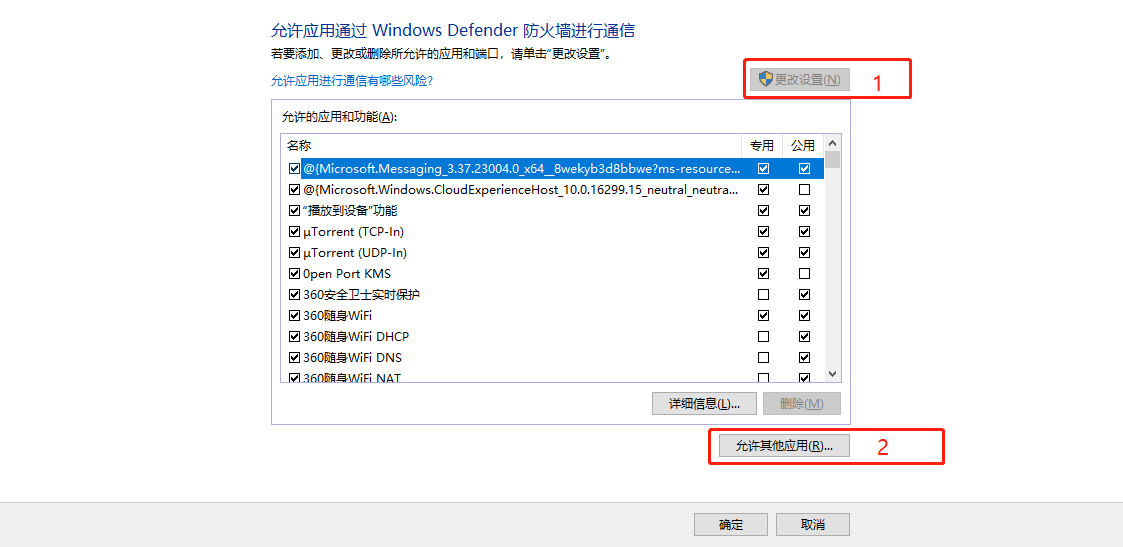5、通过弹出的界面添加matlab启动路径。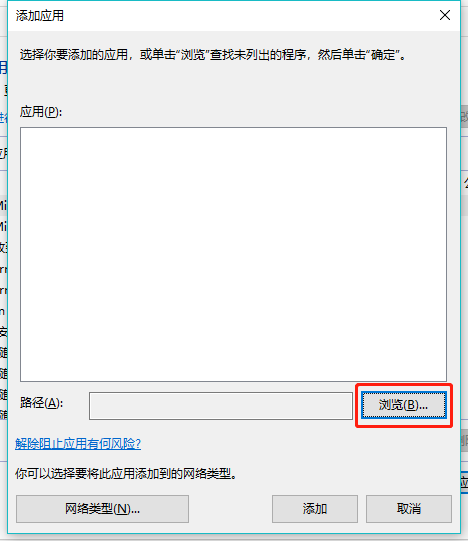6、找到matlab的启动路径，我的是D:\Program Files\MATLAB\R2017a\bin\win64，点击打开就好了。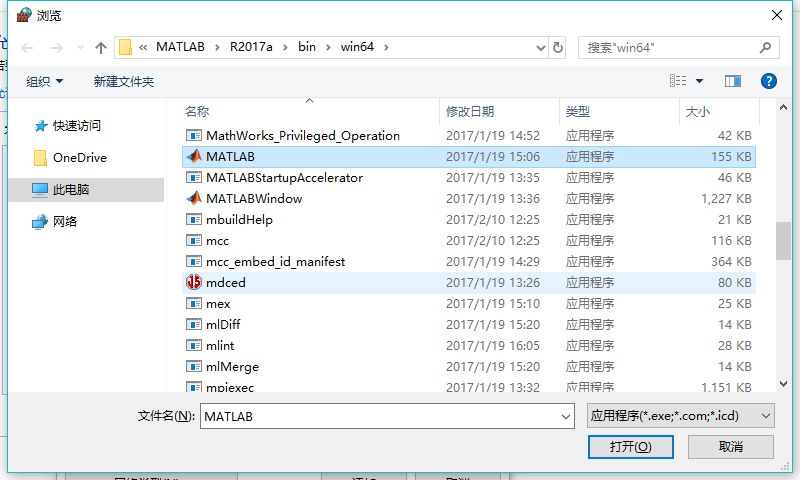7、如图已经添加好了，之后重启matlab程序就会发现打开速度变快了，而且没有错误信息显示。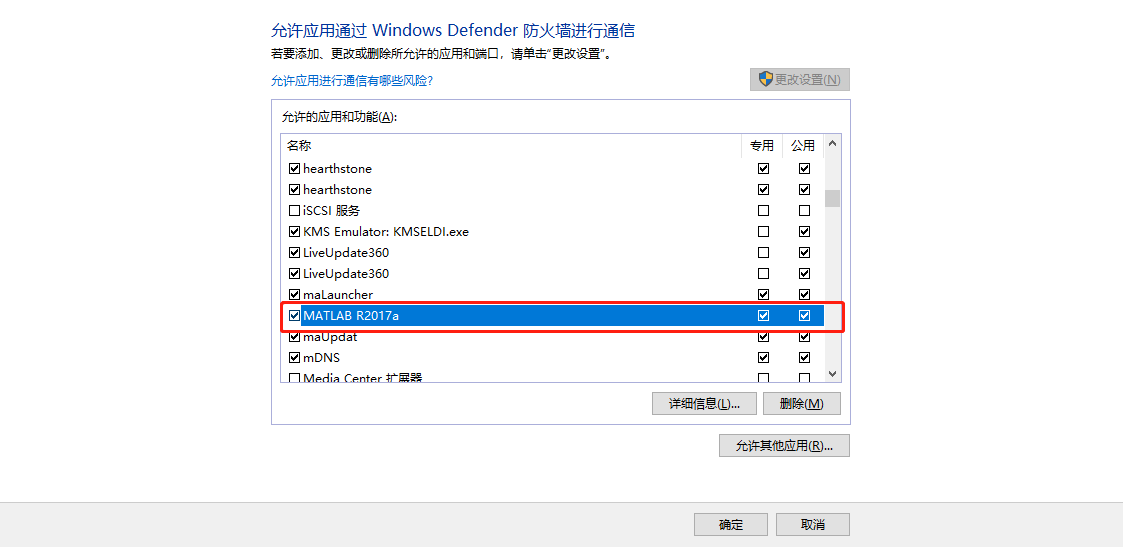展开全文• Matlab初始化太慢及相应的设置方法

万次阅读 多人点赞 2018-11-15 20:32:01
前几天装的MATLAB2018，但是初始化太慢的问题一直没解决，下面显示我遇到的问题： 等4-5分钟才就绪，真的很浪费时间，然后百度别人的解决方案，看到网上很多是文字描述，实践的截图都没几个（本人喜欢图文解答）...
• 今天终于完成安装Matlab2017b，很高兴打开软件，虽然2017b优化了实时编辑器，函数参数提醒等很多实用功能，但是初始化速度真的是等了我4-5分钟；最后找到解决办法，原因在于软件查找授权文件时间，所以解决自然...matlab matlab2017 启动慢
• Matlab R2018a初始化问题

万次阅读 多人点赞 2018-07-21 11:55:46MATLAB
• Matlab升级以后，功能的确丰富了好多，有些函数也...下面我们说说，MA TLAB初始化initializing极慢解决方法，但是可能导致Matlab启动的初始化很慢原因很多，这里我们只能对常见的集中可能的原因进行分析：一、Lic...
• 电脑重装Windows 10系统后，安装Matlab R2017b，启动时界面左下角一直显示正在初始化，并且时间，十几分钟过后，如下图所示： 在网上找了很多方法，都不行，后来看到一个博客...Matlab
• MATLAB 2017b初始化时间解决办法 最近安装了matlab 2017b，激活的时候使用了错误的文件，导致初始化时间，在网上查了很多方法都没有很好的解决问题，重装也无济于事。 最后发现把安装目录里licenses文件除了...matlab
• 为什么MATLAB报告我的变量未初始化？(Why is MATLAB reporting my variable uninitialized?)我做了一个课程，在其中一个方法中，我需要计算两点之间的距离。 所以我写了一个名为“remoteness”的普通函数来为我做这...
• MATLAB中如何初始化一个自定义长度的字符串？ 比如，我要初始化一个里面有3个0的字符串‘000’。 思路 MATLAB中是没有这样的一个函数，但是有初始化一个数组的函数，比如zeros，ones。 >> A=ones(1,3) A = ...
• 解决MATLAB一直初始化，加速MATLAB

万次阅读 多人点赞 2019-07-14 17:23:01MATLAB
• 遗传算法matlab初始化代码与论文相关的代码： 一般微分赫布学习：捕捉神经网络和大脑中事件之间的时间关系 作者：Stefano Zappacosta、Francesco Mannella、Marco Mirolli、Gianluca Baldassarre 文件树的描述 ...matlab
• 安装matlab2021b之后电脑启动变慢&&matlab初始化时间原因及解决方法matlab
• 1.1 粒子群算法建模粒子群算法首先在给定的解空间中随机初始化粒子群，待优化问题的变量数决定了解空间的维数。每个粒子有了初始位置与初始速度，然后迭代寻优。每一次迭代中，每个粒子通过跟踪两个...
• matlab导出dll及c++调用matlab初始化异常处理

千次阅读 热门讨论 2018-12-12 13:41:00
c++调用matlab的dll，折腾了一天时间，在matlab初始化时候报错“异常：0xc0000005:读取位置0x0000000000000008 时发生访问冲突”；刚开始怀疑是matlab到处的dll有问题，自己装了个matlab重新导，还是同样的错误，...
• 首先介绍一下我自己使用的有效方法，一般matlab启动初始化很慢主要的原因是在找注册文件，一般是由于安装了MAC类的软件引起的，所以我按照下文提供的方法“在【目标】中，键入如下内容”\$MATLABbinwin32MATLAB.exe”...
• Matlab R2019a初始化导致启动慢的解决方法

千次阅读 多人点赞 2019-12-18 21:29:05
Matlab R2019a初始化解决方法 导致运行很慢的主要原因是软件花费了大量的时间在寻找许可文件，具体解决方法如下： 1.右击桌面上的matlab.exe快捷方式，点击属性，画面如下： 2.目标栏: 前面是matlab.exe 的根...matlab
• initrandn() 为 Matlab 的正常（高斯）随机数生成器设置状态。 （Matlab 有两个生成器——“rand”和“randn”——... 如果可选调用参数存在但为空矩阵，或者如果用户输入为空，则会根据当前日期和时间生成默认种子。matlab
• 最近在下载新版2020MATLAB的时候发现安装好打开MATLAB后，这个位置一直在显示”正在初始化” 大概画红圈这里，因为我已经解决了，所以我这里就没了 具体解决方法就是： 首先，打开右键MATLAB图标打开属性窗口： ...windows matlab
• 加载数据加载日语元音数据集。...XTrain 中的条目是具有 12 行(每个特征一行)和不同列数(每个时间步一列)的矩阵。[XTrain,YTrain] = japaneseVowelsTrainData;[XValidation,YValidation] = japaneseVowe...
• 折腾了1.5天时间，试了n种办法才发现初始化失败的解决方法。 matlab部分： 在matlab中创建myadd.m文件： function c = myadd(a, b) c = a + b; end 步骤1：mex -setup 点击选择mex -setup C++ 步骤2：...
•   1.安装教程如下：找到Matlab点进去即可 ... 下载后解压密码：rjzkgzh 2.打开后初始化很慢： 解决方法1（如果图标在桌面）： https://blog.csdn.net/sinat_38321889/article/details/78167467 eg: D:\Ma...matlab
• 遗传算法matlab初始化代码HP 模型蛋白质折叠 在 MAI 的 CI 主题背景下开发的项目试图解决蛋白质折叠问题，以解决自回避路径约束下的优化问题，并使用所需的互补功能。 代码 它使用matlab的optimtool来支持代码的执行...
• 与传统水平集公式相比，DRLSE 的主要优点包括： 1) 它完全消除了重新初始化的需要； 2）它允许使用较大的时间步长来显着加快曲线的演化，同时确保数值精度； 3) 与传统的水平集公式相比，非常容易实现且计算效率更...matlabmatlab...

matlab初始化时间长matlab 订阅